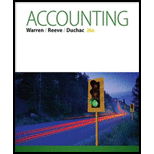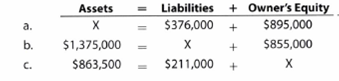Chapter 1, Problem 1.6EX### Accounting (Text Only)

26th Edition
Carl Warren + 2 others
ISBN: 9781285743615

#### Solutions

Chapter
Section### Accounting (Text Only)

26th Edition
Carl Warren + 2 others
ISBN: 9781285743615
Textbook Problem
11 views

# Accounting equationDetermine the missing amount for each of the following:To determine

Accounting equation:

Accounting equation is an accounting tool expressed in the form of equation, by creating a relation between resources or assets of a company and claims on the resources by the creditors and owners. Accounting equation is expressed as shown below:

Assets = Liabilities + Shareholders Equity

The missing amount in the given equations.

Explanation
 Asset = Liabilities + Owner's Equity Missing Value a. X = $376,000 +$895,000 $1,271,000 (1) b.$1,375,000 = X + $855,000$520,000 (2) c. $863,500 =$211,000 + X $652,500 (3) Working Note: a) Calculate the asset in the given equation. Assets = Liabilities + Owners' EquityX=$376,000 + $895,000=$1,271,000 (1)

Hence, the asset in the given equation is \$1,271,000.

b)

Calculate the liabilities in the given equation

### Still sussing out bartleby?

Check out a sample textbook solution.

See a sample solution

#### The Solution to Your Study Problems

Bartleby provides explanations to thousands of textbook problems written by our experts, many with advanced degrees!

Get Started

#### Find more solutions based on key concepts